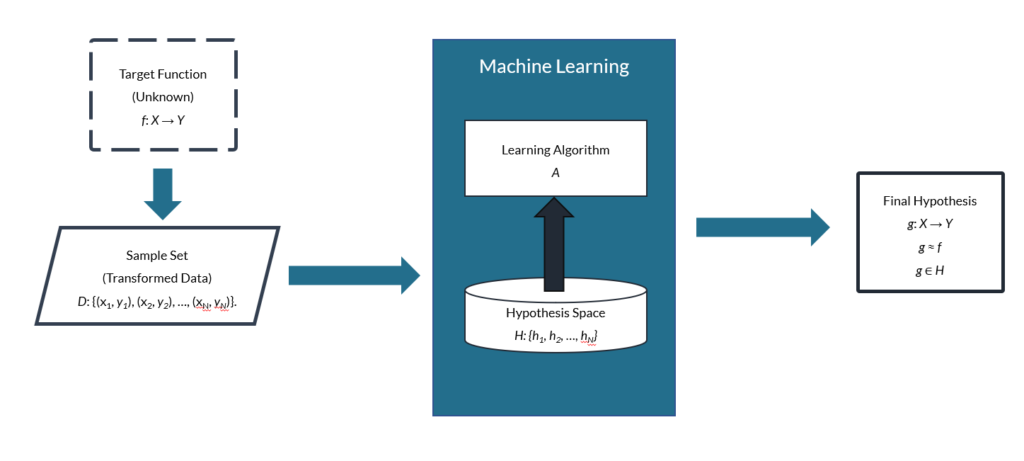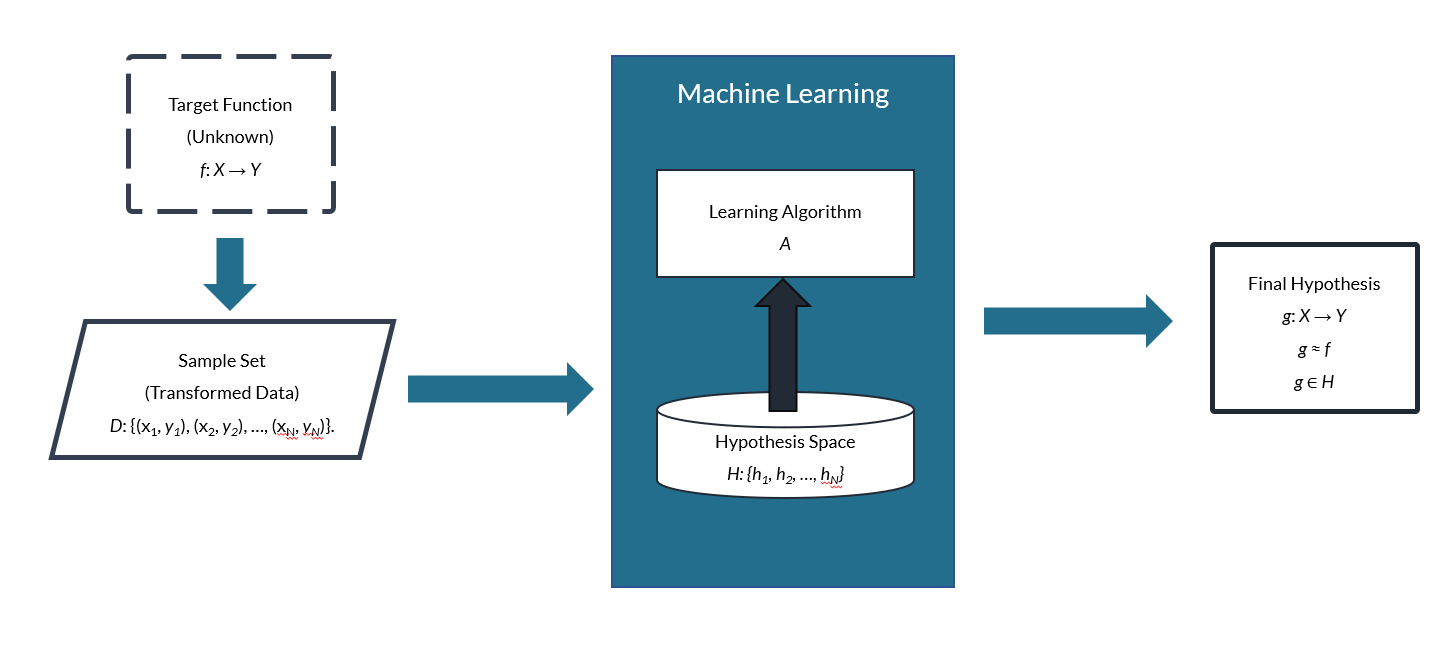# How Machine Learning Works: A Mathematical and Visual Analysis## The Purpose of Machine Learning: Approximating a Function

Machine learning techniques help software developers build customized solutions that drive significant business value. The fundamental goal of machine learning is to approximate a function that we, as software engineers, don’t know how to implement based on the available data and information.

RELATED ARTICLE: How Software Is Developed Using Machine Learning Algorithms

In any given learning problem, the function that we are trying to approximate is referred to as the target function, and we use the variable f to denote it. The function that is “learned” in place of f is referred to as the final hypothesis, and we use the variable g to denote it. This helps clarify that f and g are separate functions that can have different properties, even though g returns values close to the values that f returns for a particular data set.

## Determining a Function Within the Hypothesis Space

It is the job of a learning algorithm (A) to find the best possible function (g) for a problem. The learning algorithm does this by examining a collection of candidate functions and picking the best one. The set of functions that the learning algorithm examines is called the hypothesis space, and we use the variable H to denote it.

For example, we may determine that a neural network approach would best approximate f. That means we would utilize learning algorithms loosely modeled after biological neural networks. Dozens of artificial neural network (ANN) models exist, so H would be a set of those possible neural networks. The learning algorithm would select a specific neural network from this hypothesis space (H) as the final hypothesis (g). Any individual member of the set H is denoted as hn, so each hn is a member of (∈) the set H. In mathematical terms, then, h1H just like 1 ∈ {1, 2, 3, 4, 5}.

## Using Data to Home in on the Final Hypothesis

To find g, the learning algorithm must analyze example data. The set of examples is called the sample set, and we use the variable D to denote it. Any individual example from D can be referred to as x. In mathematical terms, D: {x1, x2, …, xN} and x1D. The total number of examples in the set is represented using the variable N.

Note that D is a subset of the domain of f. We refer to the domain of f as X, and every x in our sample set is also an element of the larger set X. We will denote the range of f as Y.

To complicate things a bit further, the composition of each x will depend on the specific problem we’re trying to solve. For example, if we’re trying to forecast weather, each x might contain a set of measurements such as time of day, location, temperature, humidity, barometric pressure, wind velocity and direction, etc. These are the features of our data, and we write them as components of a vector: x = (x1, x2, …, xd) where xi are the features. The total number of features is denoted as d. This means x is a vector of dimension d.

## The Importance of Transforming Data to Facilitate Analysis

Many learning algorithms require that X be a vector space. Often, this necessitates transforming the original data. In our weather forecasting example, the temperature, humidity, and pressure values might be real numbers; we would then need to convert the time of day and location data to real numbers as well. For example, we might convert the time of day to the number of seconds since midnight UTC and transform the location into separate latitude, longitude, and altitude values.

This can result in our transformed data having a different dimension than the original data. For practical purposes, let’s assume our sample data has been transformed so that X is a vector space.

## Delineating Input and Output Data Points

In an ideal project, output values are provided for each example, x. In this case, we denote the output value corresponding to xn as yn. In mathematical terms, then, D: {(x1, y1), (x2, y2), …, (xN, yN)}. For the purposes of data analysis, we typically assume that yn = f(xn), but this is not usually the case in the real world.

For example, weather data consists of measurements, but those measurements are only as accurate as the instruments used to make them. So, it’s more likely that yn = f(xn) + ε(xn) where ε is a function that encapsulates the measurement error and/or other “noise.” In many cases, ε will be small enough that it can be ignored, but not always.

The set Y should be a subset of the field over which X is a vector space. If we’re transforming the example data so that all the xi are real numbers, then the yn should be transformed to be real numbers as well.

## Visualizing the Machine Learning Process

To summarize, there is some unknown target function (f: X → Y) involved in converting inputs (xn) to outputs (yn), which creates a data set, D. We use a machine learning algorithm to analyze D in a specific way to find a final hypothesis (g: X → Y) that approximates f. Below, we can visualize the basic elements of a machine learning system:## Summary of Machine Learning Terminology and Variables

• Target function: f
• Final hypothesis: g
• Learning algorithm: A
• Hypothesis space: H
• An individual function within the hypothesis space: hn
• Sample set: D
• An individual example from the sample set: xn
• Total number of examples: N
• Domain of f: X
• Range of f: Y
• Individual data features: xi
• Total number of features: d
• Output values corresponding to xn: yn

## Stratus Innovations Group Can Bring the Power of Machine Learning to Your Business

The math, programming, and other work involved in machine learning can be very complex, but the practical applications are simple: increasing efficiency, creating deep insights, and driving profits for businesses of every size across industries.

When you work with Stratus Innovations Group, we do all the heavy lifting of software development, machine learning, and more so you can focus on your business and reap the benefits of cloud computing.

To speak with one of our experts about how a customized cloud solution can address your business’ needs and goals, call 844-561-6721 or fill out a simple contact form.

In the future, we’ll continue covering machine learning topics: different kinds of learning problems, how learning algorithms work, and more. So come back to our blog or let us know if you’d like to be added to our exclusive, free e-mail newsletter.

Insert math as
$${}$$